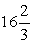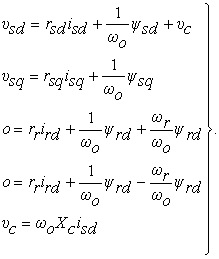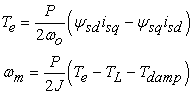﻿ Proposing New Technique for Designing AC ConvertorPublications are Open
Access in this journal
Article Versions
Export Article
• Normal Style
• MLA Style
• APA Style
• Chicago Style
Research Article
Open Access Peer-reviewed

### Proposing New Technique for Designing AC Convertor

American Journal of Electrical and Electronic Engineering. 2017, 5(3), 69-77. DOI: 10.12691/ajeee-5-3-1
Published online: May 10, 2017

### Abstract

This paper presents the tropology of direct conversion of AC-AC with step down frequency operation. Due to, the higher order harmonics AC conversion always arises difficulty and complex calculation, which effect the output response. Precise controlling and harmonics reduction is much easier using frequency control strategy, in this regards cycloconverter has drawn higher attention in terms of frequency conversion. In this paper, an approach for designing of single phase and three phase cycloconverter is reported. The gating sequence has been designed for desired frequency conversion. Again the performance of the designed converters is evaluated using Matlab/Simulink software for different types of loads. The rotor speed of Induction motor (IM) is observed here as a dynamic AC load .The complete description of the models for the frequency conversion is provided and simulation results for resistive, inductive and motor validate the developed strategy.

### 1. Introduction

Induction motor is most preferable AC machine among all other machine in industrial application due to its low cost and precise control. Several researcher has been conducted their research on ac drive and machine control. As, a result rotor current, stator flux and supply voltage control based speed control of IM is well established. However, now a day’s frequency based speed control is more preferable due to its simplicity and accurate controlling. Since, rotor speed of an induction machine is dependent on the stator pole and supply frequency, so cycloconverter is an AC-AC converter introduced to control the speed varying the frequency. A cycloconverter is a direct-frequency changer that converts ac power at one frequency to ac power at another frequency by ac-ac conversion, without an intermediate conversion link 1, 3, 5. In 1930s, phase-controlled cycloconverters with grid-controlled mercury-arc rectifiers were used in Germany to convert 3Φ 50 Hz power to 1ΦHz power for railway traction using universal motors but the fundamental guideline of this converter, conceived and patented by Hazeltine in 1926 to construct an alternating voltage wave of lower frequency from successive voltage waves of a higher frequency multiphase ac supply by a switching arrangement 2, 4. In the late 1950’s, after the development of thyristors the first new cycloconverter application were in the variable-speed constant-frequency supply systems for aircraft 6, 7. Nowadays, cycloconverterare well suited for high-power and low-speed applications, such as reversing rolling mills 8, icebreakers 9, gearless cement mills, mine hoists, ore grinding mills, Scherbius drives, ships drives etc 10. Various aspects of the cycloconverter theory and practice were discussed by McMurry 11, Gyugyi and Pelly 12 and Pelly 13 in the 1970’s.

This paper, proposed a simple control strategy for speed control of single and three phase IM using cycloconverter. For implementation used solid state semiconductor devices like as IGBT, MOSFET, SCR as switching element but IGBT is used mostly for better application 14, 15. However, IGBT performance has been taken in consideration in this paper. As it’s known that squirrel cage rotor is more preferable than wound type rotor, so all the IM was Squirrel cage type. A prototype asynchronous machine in Matlab/Simulink has been used for the simulation purpose. The improved wave shape is achieved by implementing filter circuit which is prominent in the motor speed response. To reduce harmonics from cycloconverter controlled output a LC filter circuit designed 13, 16. This paper would be appeared with the methodology of cycloconverter, frequency conversion for single and three phase system, firing sequences and result analysis for IM speed.

### 2. Methodology

I - Single phase to single phase conversion

In a single phase cycloconverter single phase input frequency is converted into single phase output. In accordance with cycloconverter theorem 1 only one side converter, either positive converter or negative converter could be activated.

Since, there is a possibility of generating of short circuit due to the at a time activation, the circuit diagram is given in Figure 1 and the current circulate either positive or negative side at a time. Activation of switching devices depends on the switching sequence. The proposed switching sequence for single phase to single phase converter is as mentioned in the following Table 1. Here 1Φ to 1Φ conversion is carried out triggering switches P1P4; P2P3; N2N3; N1N4 using S1; S2; S3; S4 gate signals respectively. Here, input signal has been taken for 0.08s where all the gating signal duration was 0.02s; however, they appear successively to form the desired output signal with an interval of 0.02s. The frequency of the output signal could be decrease using the control signal. Since, supply is 50 Hz per cycle complete in 0.02 sec and the pulse sequence design according to the time period.

• Figure 1. 1Φ to 1Φ Cycloconverter

Since, single phase is unable to develop a rotating field, in this work spit phase IM has been used with 90° phase shifting. Here, the converter is developed for reducing the frequency; however it’s possible to increase the frequency by this model. The performance of IM, synchronous speed, Ns=120f/P changes in according to the frequency changes; the speed response was analyzed with the change of frequency. As a result, d-q axis mathematical derivation has been used 17 for analyzing the speed changing of IM. The voltage equation of the motor can be written in the d-q stationary frame as follows:(1)

The equations of torque and speed are given by:(2)

Where:

ψsd, ψsq, ψrd, ψrq are the d-q- stator and rotor flux linkage, respectively,

Xsd is the d-axis stator leakage reactance,

rr is the rotor resistance,

rsd, rsq are the rotor resistance,

ωm is the base speed, 314 rad/sec,

ωr is the rotor speed, electric rad/sec,

Te is the developed torque,

Tdamp is the damping torque,

P is the number of poles.

II - Three phase to single phase conversion

The three-phase input cycloconverter is identical to the single phase input cycloconverter except that there are three input voltages which have 120° shift between each other instead of single phase 180°. Two three phase full-wave (six-pulse) bridge converters (rectifier), positive and negative are connected back to back. For each bridge six switches are used. In Figure 2 the schematic diagram of three phase to single phase cycloconveter presented 1 where current flow only one converter. The frequency of the output signal could be decrease using the control signal proposed in Table 2. Here 3Φ to 1Φ conversion, gating signals S1, S2, S3 has been given to the combination P1P4; P3P6; P2P5 respectively for positive cycle conversion. On the other hand, S4, S5, S6 provided to the combinations N1N4; N3N6; N2N5 respectively for negative cycle conversion. As, the supply signal taken for 0.08s and total 12 switches are used, so every switch will be activated for around 0.066s according to the Table 2 on-off sequence. Both frequency and phase conversion is possible to convert using the given circuit.

• Figure 2. 3Φ to 1Φ Cycloconverter

III - Three phase to three phase conversion

Three identical three phase input to single phase output with 3 pulse cycloconverter connected together to a three phase supply load. A Cycloconverter consist of 36 switches to generate the firing pulse. In Figure 3 the schematic diagram of three phase Cycloconverter presented where current circulating either positive or negative because both sides do not conduct at the same time. In three phase cycloconverter frequency has been decreased using the control signal proposed in Table 3, Table 4 and Table 5. As 3Φ - 3Φ is a cascaded network of three 3Φ - 1Φ phase circuit, so three 3Φ - 1Φ circuits are used for 3Φ - 3Φ. Moreover, total 18 gating sequence were used to conduct current in three separate circuits, where time duration and sequence of individual switch kept same as 3Φ -1Φ converter. However, most important fact that, 6 pulses of one circuit is 120° or 0.02s phase shifted from each other in order to generate 120° shifted three phase output; the on-off time for three circuit has been presented in Table 3, Table 4 and Table 5. LC filter circuit designed to reduce harmonics from cycloconverter controlled output voltage where using Induction Motor.

• Figure 3. 3Φ to 3Φcycloconverter

### 3. Simulation and Result

Matlab/ Simulink is worldwide recognized software for it compatibility and accuracy. In this paper, all the simulation has been performed using Matlab/ Simulink. In Figure 4, Figure 5, Figure 6 Simulink model of 1Φ to 1Φ, 3Φ to 1Φ and 3Φ to 3Φ converter has been constructed with Induction Motor according to schematic Figure 1, Figure 2 and Figure 3 respectively and IGBT was used as switching components. Other switching components like as SCR, MOSFET has been tasted. However, IGBT provides better outputs because on-state current density, low driving power and a simple drive circuit due to the input MOS gate structure. Simulink prototype has been used to observe the speed variations. The pulse train has been developed according the constructed Table 1, Table 2, Table 3, Table 4 and Table 5. The supply voltage and frequency was considered 220V and 50 Hz respectively. In 3Φ-1Φ conversion 1Φ load has been used to observe the frequency conversion. Besides, 3Φ - 3Φ is a none other than a cascaded network of three 3Φ - 1Φ phase circuit. The complexity arises in 3Φ - 3Φ during the pulse sequence generation which is presented in Table 3, Table 4 and Table 5. Three phase squirrel cage IM was used despite of single phase IM in Figure 6. The detailed Simulink model for 3-3 is comparatively prodigal, so subsystems are suitable for the construction. As our target model has been developed for frequency step down purpose, it is possible to make it step up using same construction where only triggering sequence requires alternations.

In Figure 7 the output wave form of voltage and current has been observed where the frequency has been stepped down to half at time instant 0.04s. Moreover, using the same principle frequency has been reduced to one third for three phase to single phase conversion which is presented in Figure 8. The output frequency for three phase to three phase cycloconverter has been presented in Figure 9 where, at time 0.08s frequency reduced one third to its supply frequency. However, it is possible to change the frequency at any ratio only changing the switching patterns using same network. Here, output voltage and current are phase shifted with prominent presence of harmonics. In single phase cycloconverter the load has been changed to verify the effect of inductance and resistance in terms of their voltage and current response. It is seen in Figure 10 and Figure 11 that both inductive and resistive load has identical voltage response. On the other hand, voltage and current in inductive load is dramatically high with some abnormal transit. Since synchronous speed Ns=120f/p, here in Figure 12, Figure 13 and Figure 14 the response of in speed of induction motor has been observed, where the speed has been reduced according to the frequency reduction. It’s quite clear that, the speed fluctuation in three phase motor is lager compared to single phase motor due to the harmonics effect, and proper selection of filtering circuit might be a solution of this unexpected phenomenon. Since torque is a function of flux and current and inversely proportional to speed. So torque has been increased. Torque is varying with time and rotor speed and maximum voltage can be achieved more quickly and get stable in a specific range and so on. If magnetic field completely develop before 0.025 sec, the fluctuation between speed and torque which can be control.Torque characteristics of the induction motor that matches with demand torque characteristic by the use of cycloconverter and its control circuit at the different desire frequency.

• Figure 4. 1-Φ to 1-Φ cycloconverter with Induction Motor
• Figure 5. 3-Φ to 1-Φ cycloconverter with Induction Motor
• Figure 6. 3-Φ to 3-Φ cycloconverter with Induction Motor
• Figure 7. Voltage and Current output waveform of 1Φ to 1Φ Cycloconverter
• Figure 8. Voltage and Current output waveform of 3Φ to 1Φ Cycloconverter
• Figure 9. Voltage and Current output waveform of 3Φ to 3Φ Cycloconverter
• Figure 10. Resistive and Inductive Load Effect of 1Φ to 1Φ Cycloconverter
• Figure 11. Resistive and Inductive Load Effect of 3Φ to 1Φ Cycloconverter
• Figure 12. Rotor speed response and Electromagnetic Torque at 50Hz and 25Hz for 1Φ to 1Φ cycloconverter
• Figure 13. Rotor speed response and Electromagnetic Torque at 50Hz and 16.67Hz for 3Φ to 1Φ cycloconverter
• Figure 14. Rotor speed response and Electromagnetic Torque at 50Hz and 16.67Hz for 3Φ to 3Φ cycloconverter

### 4. Conclusion

Generally the operation of converters and maintenance needs expertise and skilled manpower. But as proposed in this paper generalized functionality of cycloconverter reduces the need for new converter hardware. The use of cycloconverter in the future reduces the need for learning many varying converter topologies. In this paper, cyloconverter circuits are designed and simulated and desired results are obtained. Three phase cycloconverter used for three phase motor to generate supply torque characteristics that matches with demand torque characteristics of particular machine by the use of designing cycloconverter different desired frequency are obtained to equalize the torque demand of machine. However, High number of pulses will not complicate the control circuits, because it will just increase the number of these control circuits. Increasing number of pulses will improve the cycloconverter output waveforms and will decrease the harmonic component values. After modification of filter circuit, torque and speed characteristics of induction motor at the time of changing frequency is improved; further simulations are also carried out with simple RL load. An undesirable spike seems to appear with a reasonable degree of magnitude that requires elimination. This probably could be solved using novel control strategies that could be investigated in future.

### References

  Muhammad H. rashid. Power Electronics Circuit, Devices and Applications. Prentice Hall Of India, Third Edition; 2007. In article  Bosh Bimal k. Modern Power Electronics and AC Drives. Prentice Hall PTR; 2002. In article  Vodovozov Valery. Introduction to Power Electronic.1stedition. In article  Sharma Anshu, Sisodiya Shilpi. A Survey on Single Phase to Three Phase Cyclo-Converter Fed Induction Motor. International Journal for Scientific Research & Development, 2015; Vol. 3, Issue 10. In article  LiuYazhou, Heydt G.T, ChuR. F. The power quality impact of cycloconverter control strategies. IEEE Transactions on Power Delivery, April 2005; Volume: 20, Issue: 2. In article View Article  Chirgwin K.M, Stratton L.J. Variable-speed constant-frequency generator system for aircraft. AIEE Trans. Appl. Ind., 1959; vol. 78, pt II, pp. 304-310. In article View Article  Mamoun A. Development of cycloconverter. Canadian Conference on Electrical and Computer Engineering, 2003. IEEE CCECE 2003 May 4-7, Montreal. Vol. 1, pp. 521-524. In article View Article  HagmannR.AC-cycloconverter drives for cold and hot rolling mill applications. IEEE IAS Conf., 1991; vol. 2, pp. 1134-1140. In article View Article  HillW. A, CreelmanG, MischkeL. Control strategy for an ice breaker propulsion system. IEEE Transactions on Industry Applications , Jul/Aug 1992; Volume: 28, Issue: 4. In article View Article  Stemmler Herbert. High-power industrial drives. Proceedings of the IEEE, Aug 1994; Volume: 82, Issue: 8, pp. 1266-1286. In article View Article  McMurry W. The Theory and Design of Cycloconverters. England: MIT Press, 1972. In article View Article  Gyugyi L, Pelly B.R. Static Power Frequency Changers. New York, USA: Wiley-Interscience, 1976. In article  Pelly B.R. Thyristor phase controlled converters and cycloconverters: operation, control and performance. Wiley-interscience, New York 1971; 3rd Edition. In article  Govil Vinamra Kumar, Chaurasia Yogesh. Modelling & Simulation of PWM Controlled Cycloconverter FED Split Phase Induction Motor. International Journal of Advanced Research in Electrical, Electronics and Instrumentation Engineering, September 2012; Vol. 1, Issue 3. In article  Ahmed Iftakher and Khan Md. Shibli Nomani. Designing And Analysis Of Cycloconverter To Run Variable Frequency Drive Motor. International Journal of Technology Enhancements and Emerging Engineering Research.vol 1, issue 4, issn 2347-4289. In article View Article  Negalur Keshav B, Joshikulkarni A.S. Simulation Of 3-Ph To 3-Ph Cycloconverter Fed Variable Speed Drive. International Journal of Engineering Trends and Technology (IJETT), May 2013; Volume4 Issue5. In article View Article  Singh Abhishek Pratap, Giri V.K. Modeling and Simulation of Single Phase Cycloconverter. International Journal of Engineering Science & Advanced Technology. Volume-2, issue-2, 346-351. In article View ArticleThis work is licensed under a Creative Commons Attribution 4.0 International License. To view a copy of this license, visit http://creativecommons.org/licenses/by/4.0/